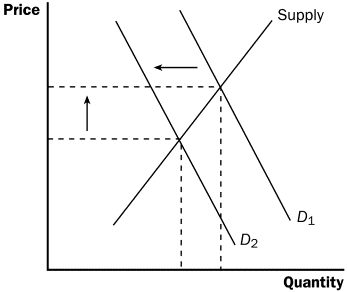# Price Elasticity of Supply

52

PRICE ELASTICITY OF SUPPLY
The relative response of a change in quantity supplied to a relative change in price. More specifically
the price elasticity of supply can be defined as the percentage change in quantity supplied due to a percentage change in supply price.
• Calculating elasticities between two points at the same curve involves arc elasticity method.

• While calculating elasticity at a certain point involves point elasticity method. Virtual UniversityDETERMINANTS OF PRICE ELASTICITY OF SUPPLY
• If costs increases, lower will be the supply. Lower the costs the more will be the supply.
• Amount of time given to quantity respond to a price increase or decrease. There may be
immediate time period, short term and long term time period.